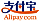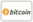Bookcover of Boundary value problems for nonlinear ordinary differential equation
Booktitle:

# Boundary value problems for nonlinear ordinary differential equation

Editions universitaires europeennes (2016-08-12 )eligible for voucher
ISBN-13:

### 978-3-639-73758-5

ISBN-10:
363973758X
EAN:
9783639737585
Book language:
English
Blurb/Shorttext:
The objective of the book is devoted to the study of the existence of solutions for some boundary value problem generated by the fourth and fifth order nonlinear differential equations. In the first three results, we establish the existence of positives solutions for nonlinear fifth-order differential equations with two and three points boundary conditions by using some fixed points theorems notably, the Schauder-theorem, Guo-Krasnosel'skii theorem on expansion and compression of cones and the upper and lower technical solutions. In the fourth chapter, we establish the existence of multiple positive solutions for a system of nonlinear second-order three point boundary value problem by using the Leggett-Williams fixed point theorem.In the last chapter, we are interested the existence of solutions and multiple solutions for differential fourth-order differential equation with deviating arguments by using the Schauder fixed point theorems, the concept of the topological degree and the method of upper and lower solutions.
Publishing house:
Editions universitaires europeennes
Website:
http://www.editions-ue.com/
By (author) :
Naceri Mostepha
Number of pages:
96
Published on:
2016-08-12
Stock:
Available
Category:
Mathematics
Price:
32.90 €
Keywords:
Schauder xed point theorem, Leggett-Williams xed point, Guo-Krasnosel'skii xed point theorem in cone, Green function, upper and lower solutions, topological degree, dierential equations of fth and fourth-order, systems### Categories